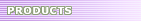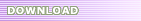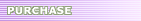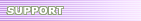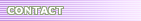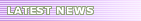# Ellemtel C++ Style and Rules - Expressions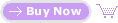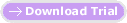15. Expressions Rec. 56Use parentheses to clarify the order of evaluation for operators in expressions. There are a number of common pitfalls having to do with the order of evaluation for operators in an expression. Binary operators in C++ have associativity (either leftwards or rightwards) and precedence. If an operator has leftwards associativity and occurs on both sides of a variable in an expression, then the variable belongs to the same part of the expression as the operator on its left side. In doubtful cases, parentheses always are to be used to clarify the order of evaluation. Another common mistake is to confuse the assignment operator and the equality operator. Since the assignment operator returns a value, it is entirely permitted to have an assignment statement instead of a comparison expression. This, however, most often leads straight to an error. C++ allows the overloading of operators, something which can easily become confusing. For example, the operators << (shift left) and >> (shift right) are often used for input and output. Since these were originally bit operations, it is necessary that they have higher priority than relational operators. This means that parentheses must be used when outputting the values of logical expressions. Example 62: Problem with the order of evaluation ``` // Interpreted as ( a= b && c < d && e + f <= g + h; // No! int j = ( a >= b ) && ( c < d ) && (( e + f ) <= ( g + h )); // Better ```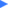Don't waste time on clarify C++ expressions yourself,  use SourceFormatX C++ Code Formatter to do it for your team.